# MSBSHSE Solutions For Class 9 Maths Part 1 Chapter 6 Financial Planning

MSBSHSE Solutions For Class 9 Maths Part 1 Chapter 6 Financial Planning is one of the best ways to strengthen one’s skills and knowledge. It contains all the relevant study material that can help the students score well in the examinations. Students can easily download the solutions in PDF format for free. A proper understanding of this chapter will further help the students with a few other chapters in higher classes as well. The solutions are provided by the subject experts and are accurate. Every question is explained stepwise for a better understanding of the students. They can refer to these solutions for reference purposes. These solutions facilitate students to create good knowledge about the basic concepts of Mathematics. This chapter of MSBSHSE Solutions for Class 9 is based on computation of income tax. Some of the essential topics of this chapter are listed below.

• Savings
• Investments
• Levying of taxes or Taxation
• Utility of taxes
• Types of taxes
• Income tax
• Computation of income tax

## Download the PDF of Maharashtra Solutions For Class 9 Maths Part 1 Chapter 6 Financial Planning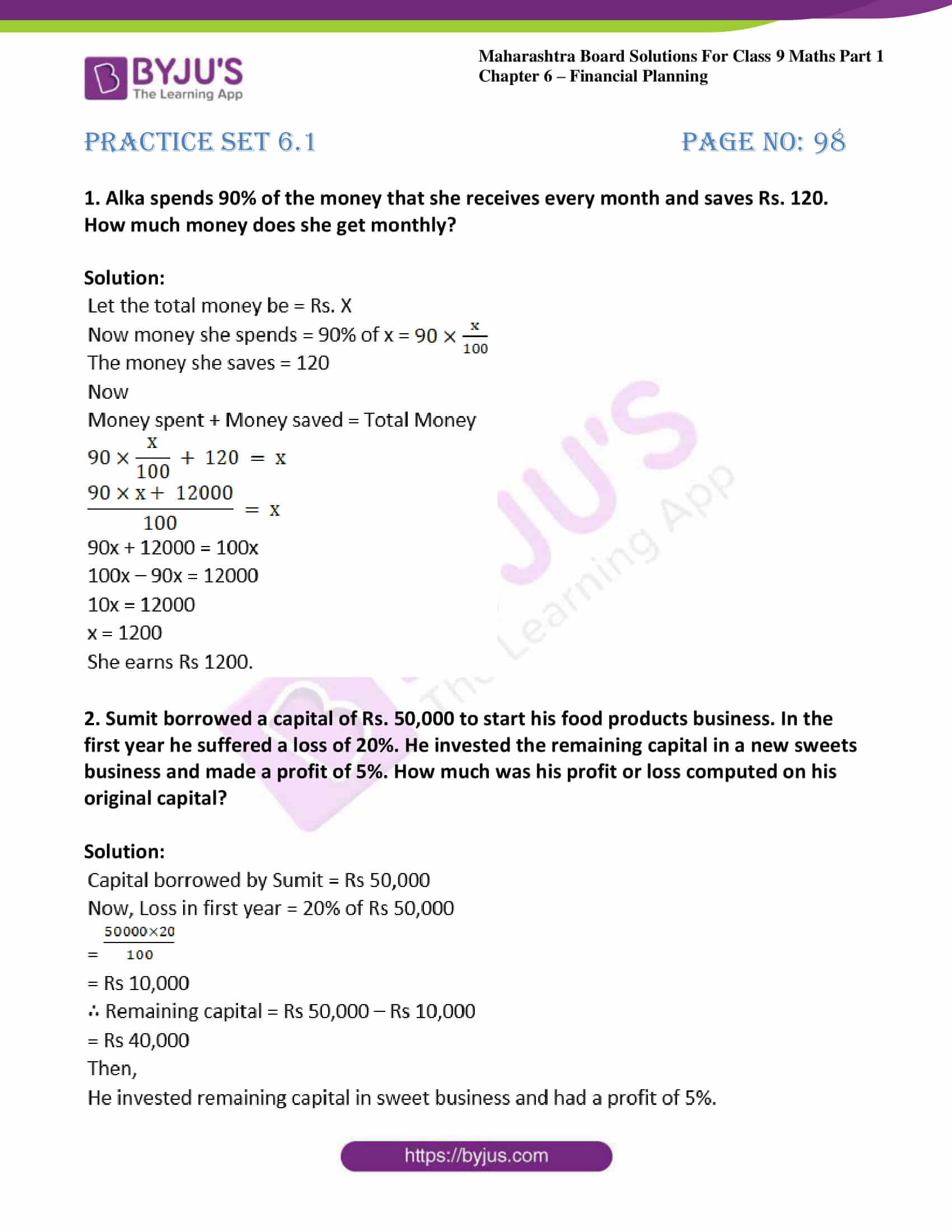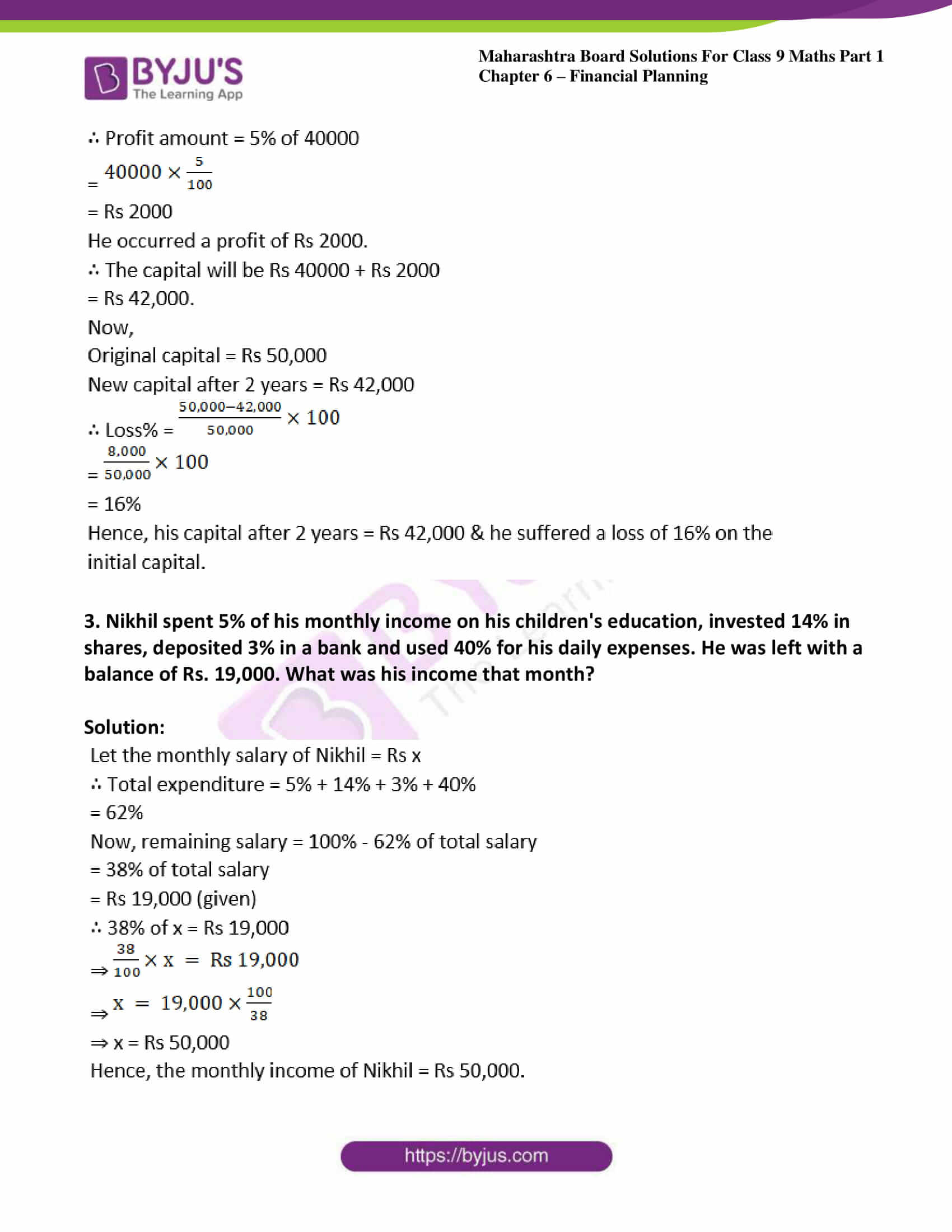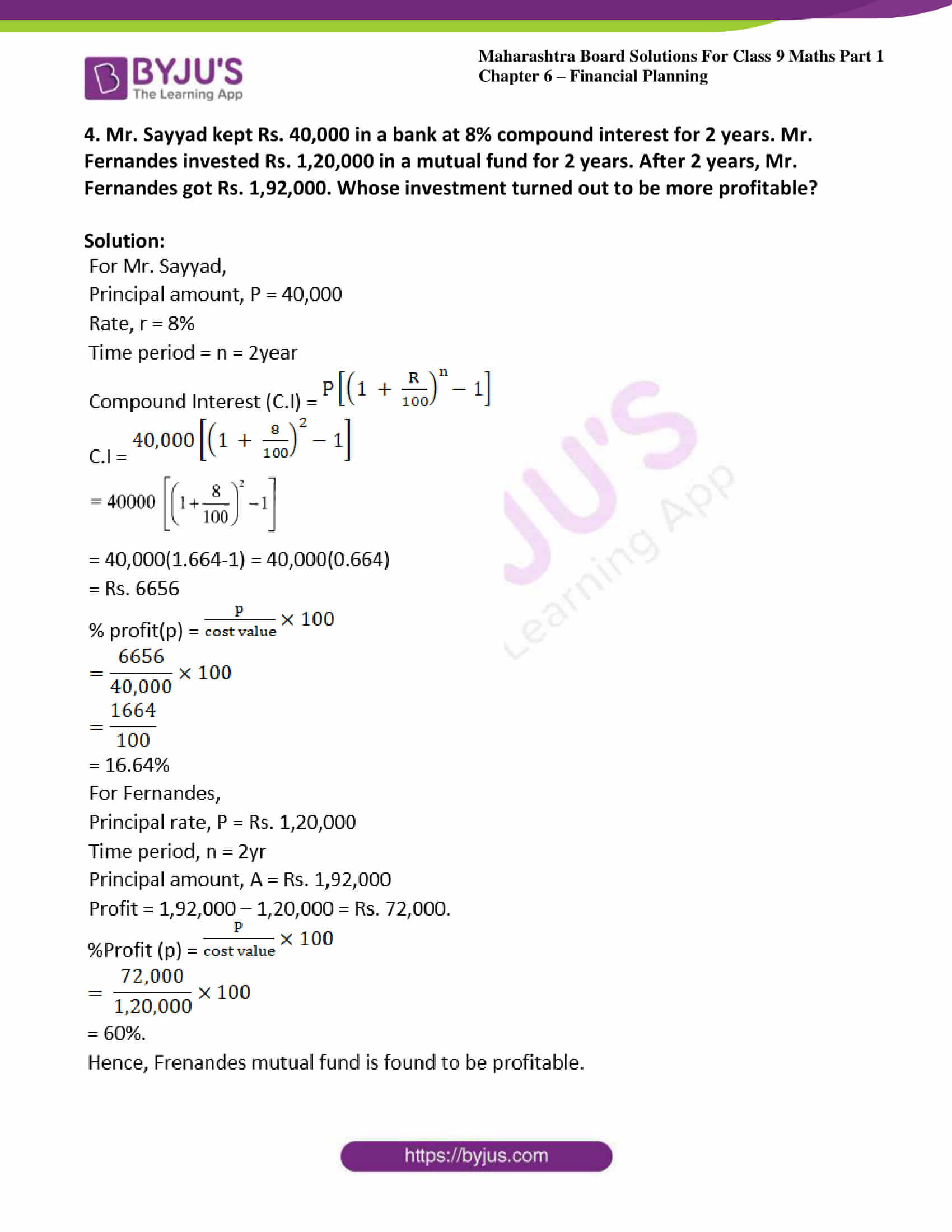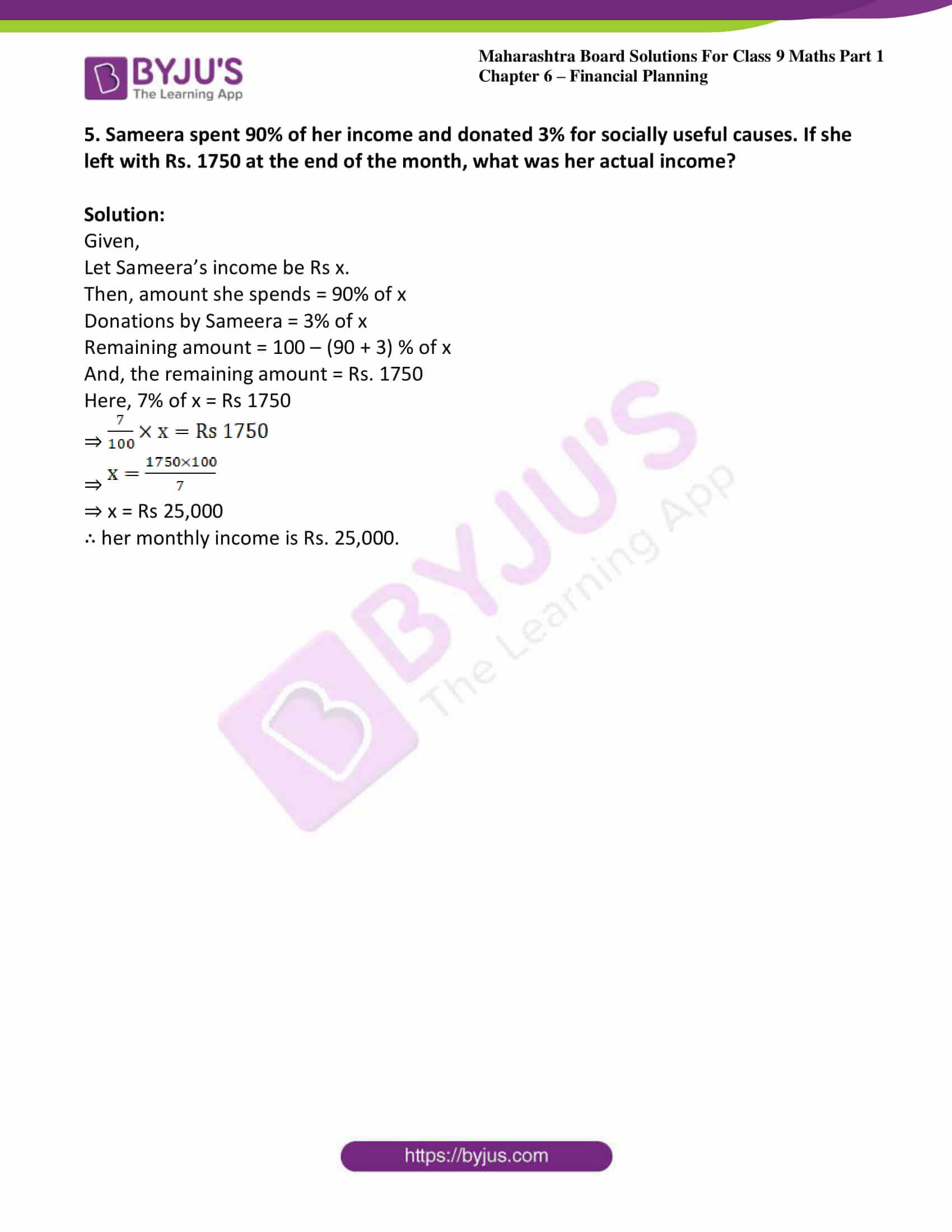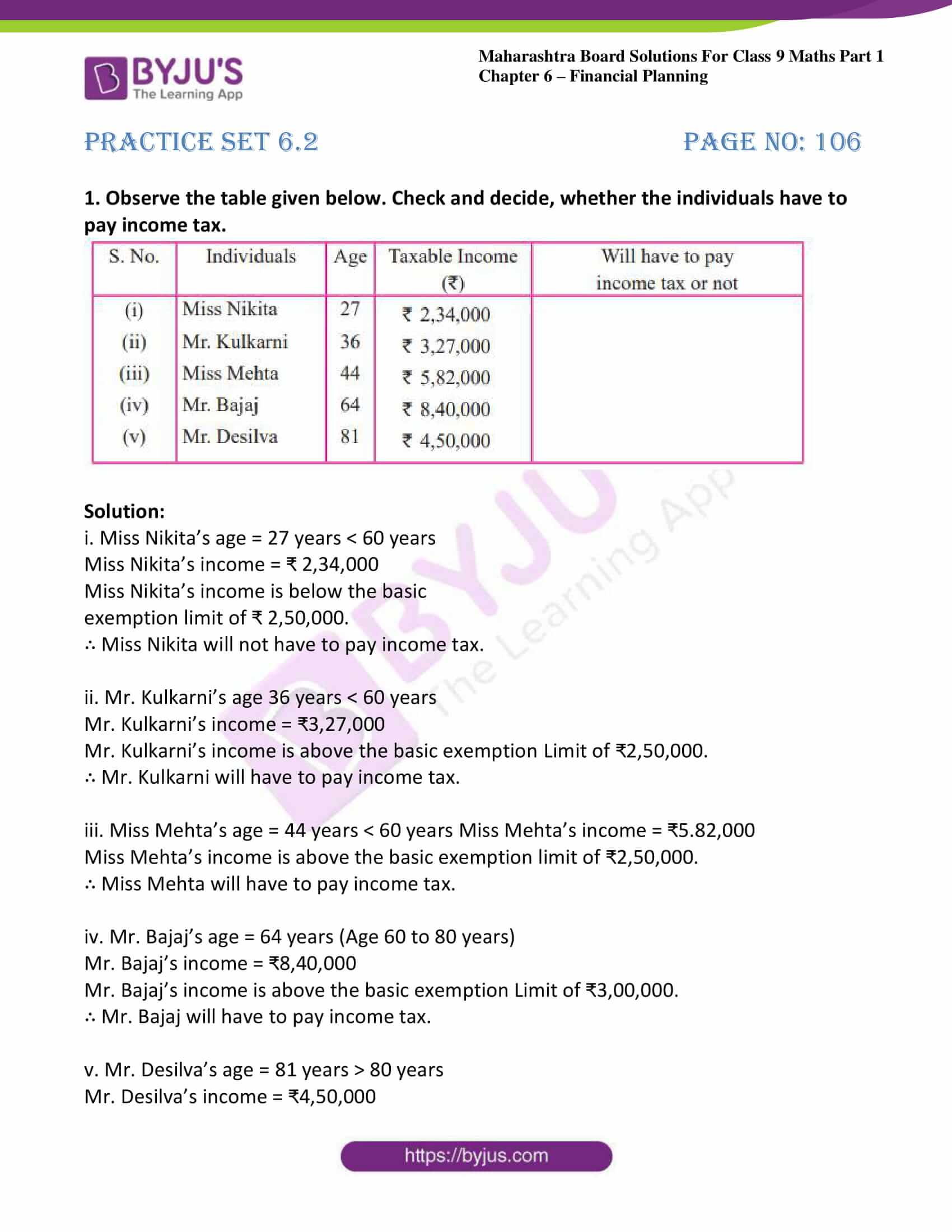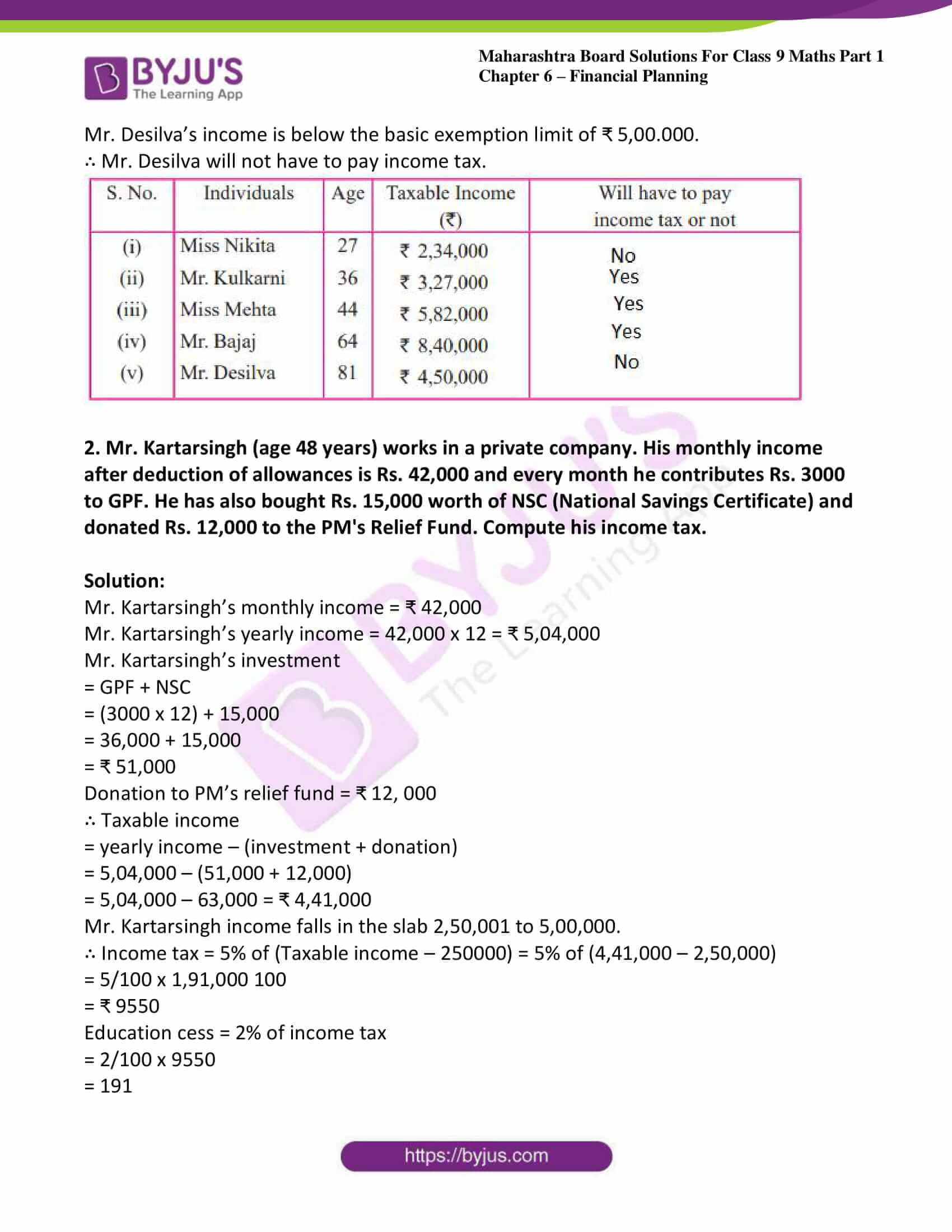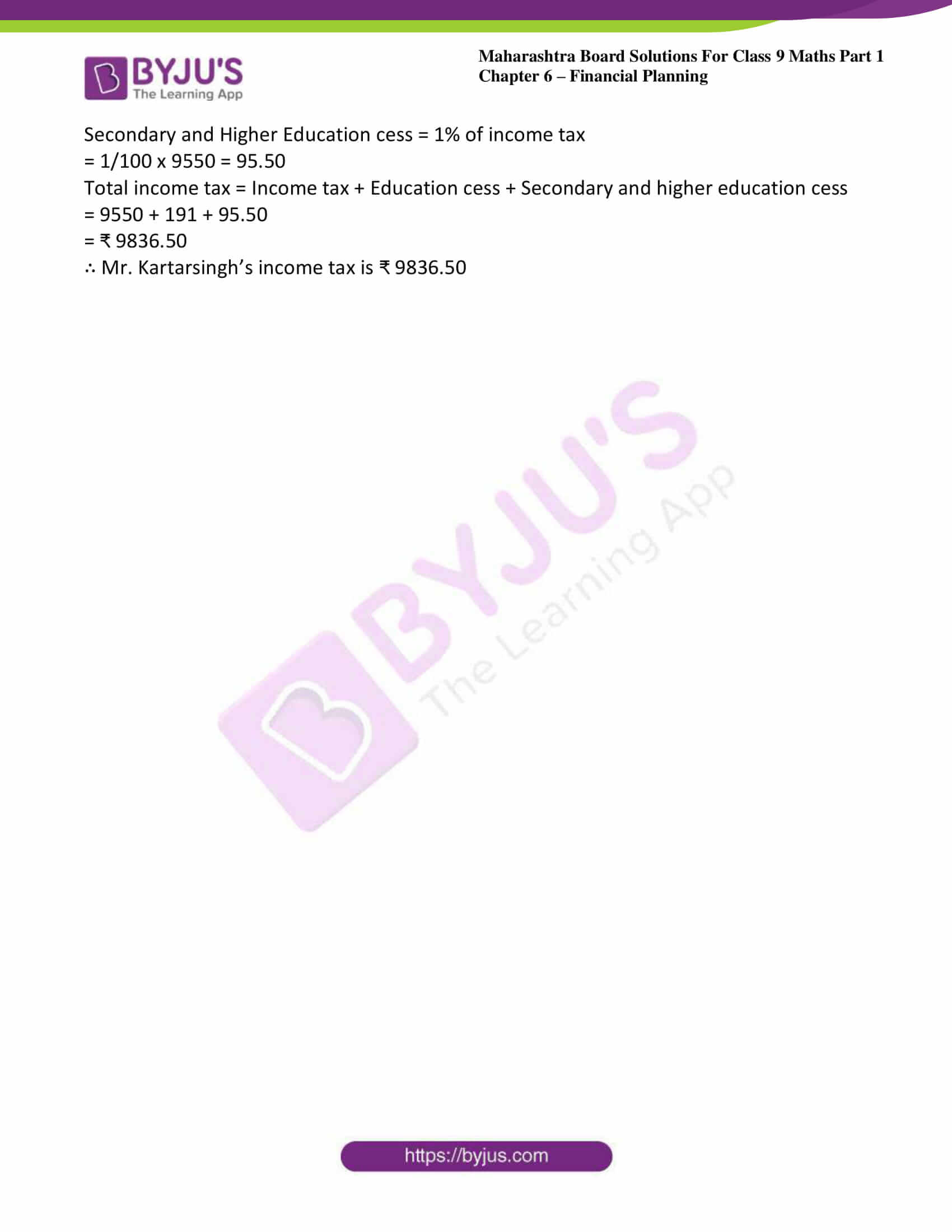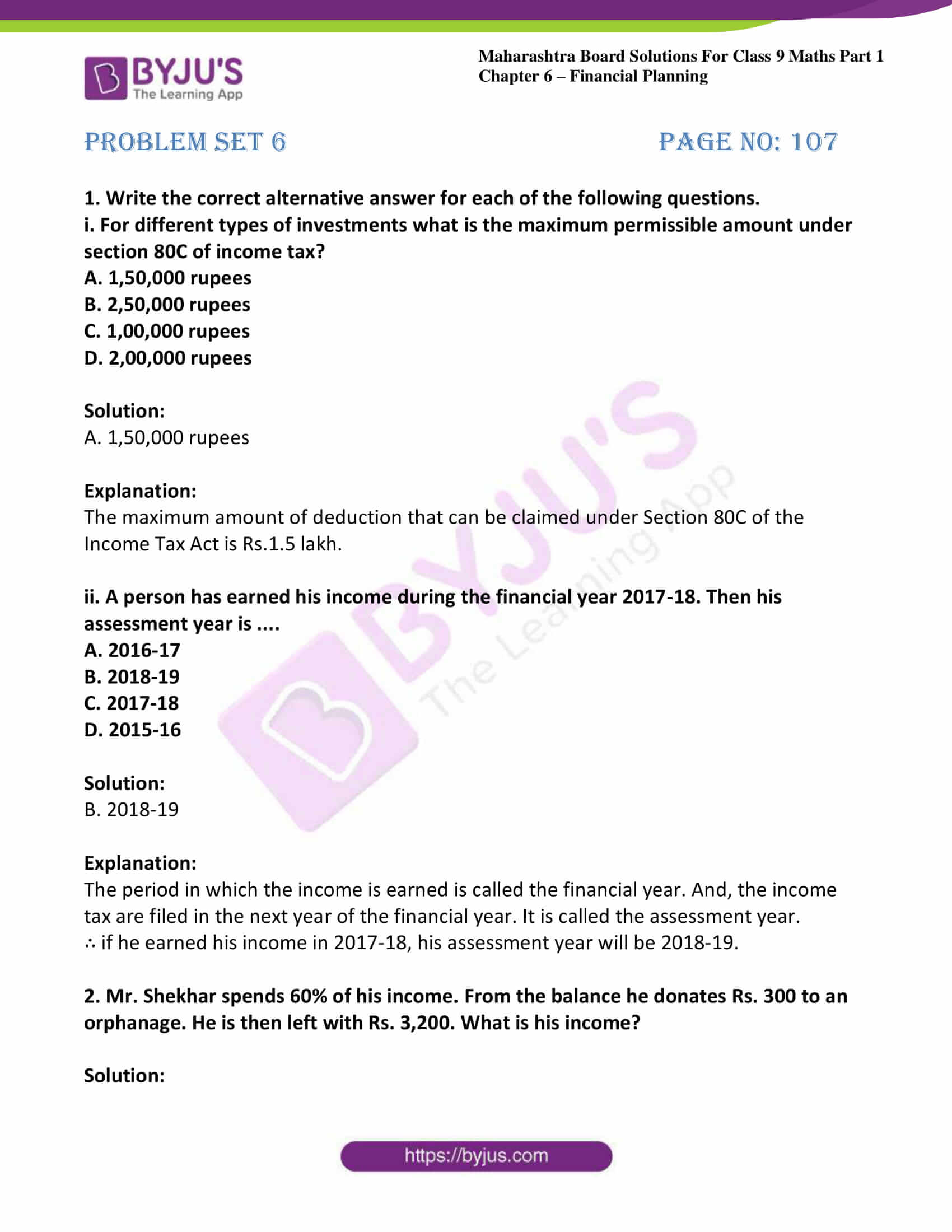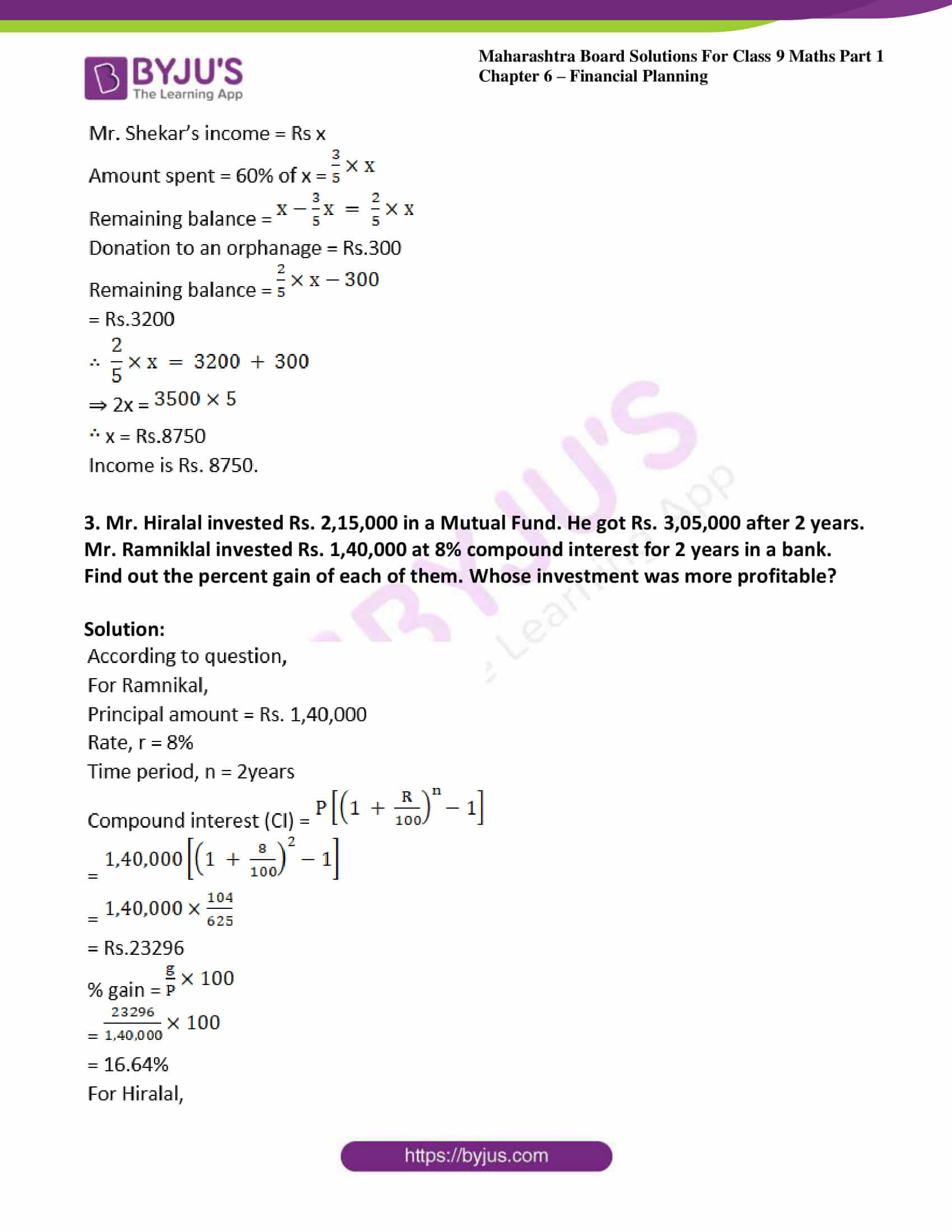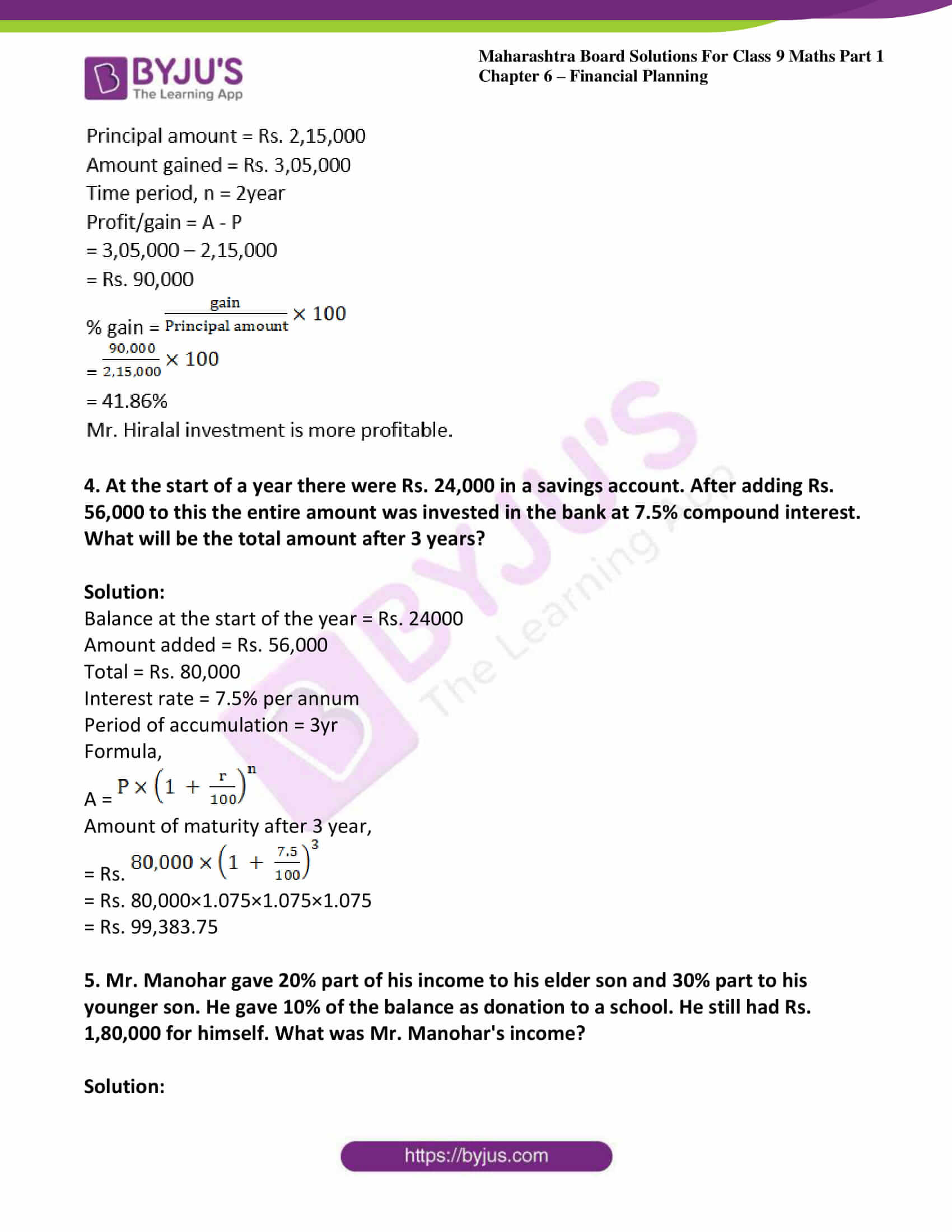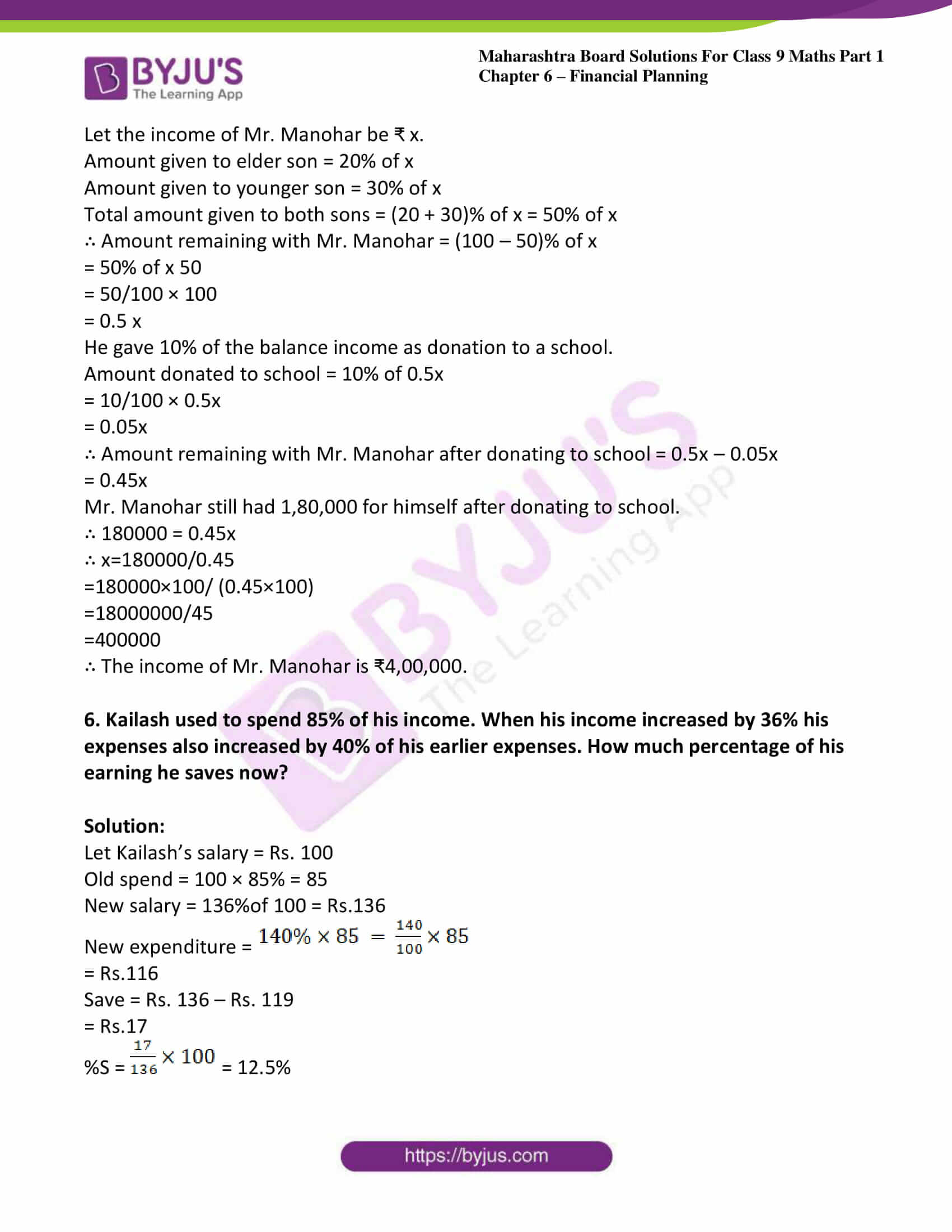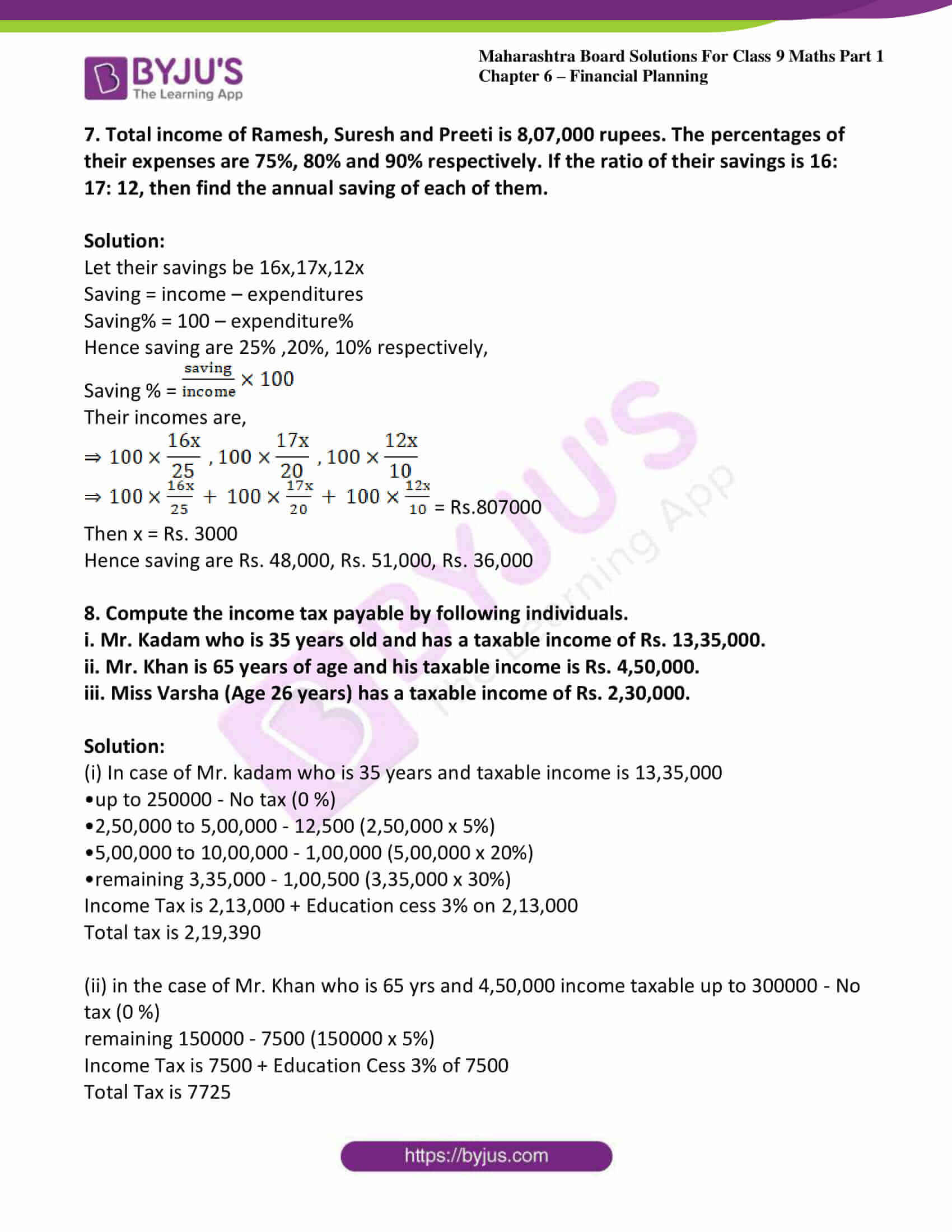### Access answers to Maths MSBSHSE Solutions For Class 9 Part 1 Chapter 6 – Financial Planning

Practice set 6.1 Page no: 98

1. Alka spends 90% of the money that she receives every month and saves Rs. 120. How much money does she get monthly?

Solution: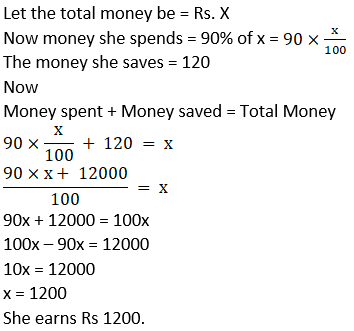2. Sumit borrowed a capital of Rs. 50,000 to start his food products business. In the first year he suffered a loss of 20%. He invested the remaining capital in a new sweets business and made a profit of 5%. How much was his profit or loss computed on his original capital?

Solution: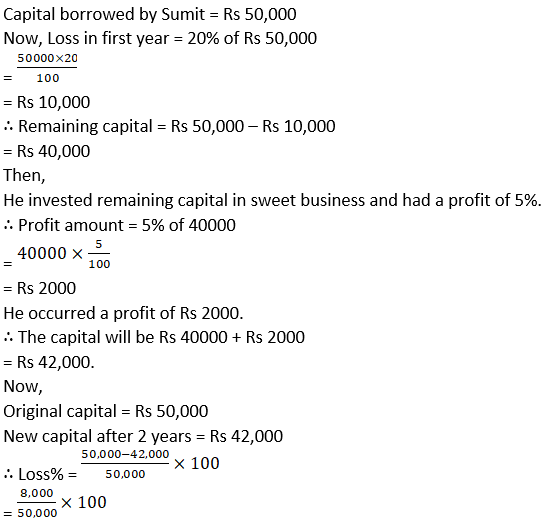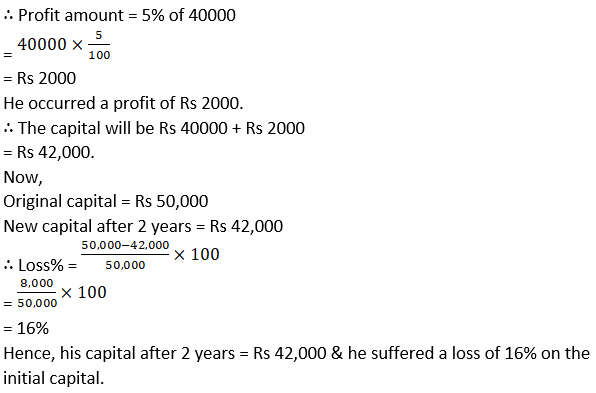3. Nikhil spent 5% of his monthly income on his children’s education, invested 14% in shares, deposited 3% in a bank and used 40% for his daily expenses. He was left with a balance of Rs. 19,000. What was his income that month?

Solution: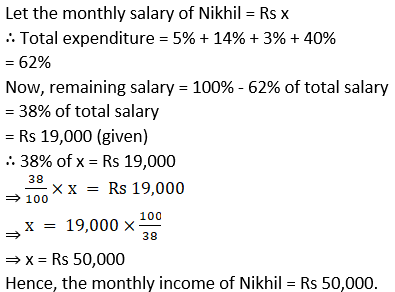4. Mr. Sayyad kept Rs. 40,000 in a bank at 8% compound interest for 2 years. Mr. Fernandes invested Rs. 1,20,000 in a mutual fund for 2 years. After 2 years, Mr. Fernandes got Rs. 1,92,000. Whose investment turned out to be more profitable?

Solution: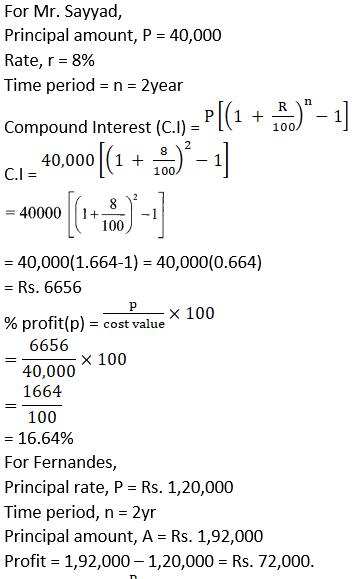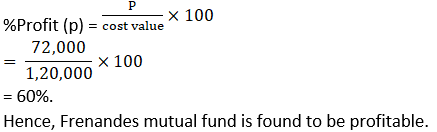5. Sameera spent 90% of her income and donated 3% for socially useful causes. If she left with Rs. 1750 at the end of the month, what was her actual income?

Solution:

Given,

Let Sameera’s income be Rs x.

Then, amount she spends = 90% of x

Donations by Sameera = 3% of x

Remaining amount = 100 – (90 + 3) % of x

And, the remaining amount = Rs. 1750

Here, 7% of x = Rs 1750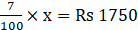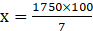⇒ x = Rs 25,000

∴ her monthly income is Rs. 25,000.

Practice set 6.2 Page no: 106

1. Observe the table given below. Check and decide, whether the individuals have to pay income tax.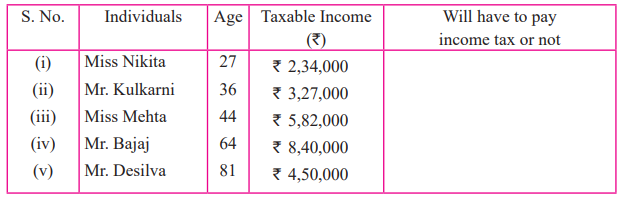Solution:

i. Miss Nikita’s age = 27 years < 60 years

Miss Nikita’s income = ₹ 2,34,000

Miss Nikita’s income is below the basic

exemption limit of ₹ 2,50,000.

∴ Miss Nikita will not have to pay income tax.

ii. Mr. Kulkarni’s age 36 years < 60 years

Mr. Kulkarni’s income = ₹3,27,000

Mr. Kulkarni’s income is above the basic exemption Limit of ₹2,50,000.

∴ Mr. Kulkarni will have to pay income tax.

iii. Miss Mehta’s age = 44 years < 60 years Miss Mehta’s income = ₹5.82,000

Miss Mehta’s income is above the basic exemption limit of ₹2,50,000.

∴ Miss Mehta will have to pay income tax.

iv. Mr. Bajaj’s age = 64 years (Age 60 to 80 years)

Mr. Bajaj’s income = ₹8,40,000

Mr. Bajaj’s income is above the basic exemption Limit of ₹3,00,000.

∴ Mr. Bajaj will have to pay income tax.

v. Mr. Desilva’s age = 81 years > 80 years

Mr. Desilva’s income = ₹4,50,000

Mr. Desilva’s income is below the basic exemption limit of ₹ 5,00.000.

∴ Mr. Desilva will not have to pay income tax.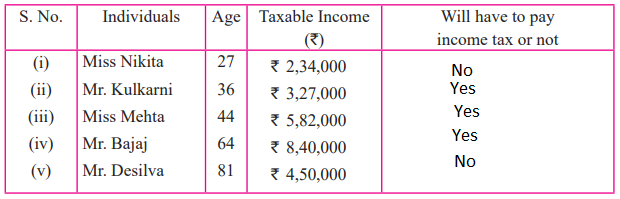2. Mr. Kartarsingh (age 48 years) works in a private company. His monthly income after deduction of allowances is Rs. 42,000 and every month he contributes Rs. 3000 to GPF. He has also bought Rs. 15,000 worth of NSC (National Savings Certificate) and donated Rs. 12,000 to the PM’s Relief Fund. Compute his income tax.

Solution:

Mr. Kartarsingh’s monthly income = ₹ 42,000

Mr. Kartarsingh’s yearly income = 42,000 x 12 = ₹ 5,04,000

Mr. Kartarsingh’s investment

= GPF + NSC

= (3000 x 12) + 15,000

= 36,000 + 15,000

= ₹ 51,000

Donation to PM’s relief fund = ₹ 12, 000

∴ Taxable income

= yearly income – (investment + donation)

= 5,04,000 – (51,000 + 12,000)

= 5,04,000 – 63,000 = ₹ 4,41,000

Mr. Kartarsingh income falls in the slab 2,50,001 to 5,00,000.

∴ Income tax = 5% of (Taxable income – 250000) = 5% of (4,41,000 – 2,50,000)

= 5/100 x 1,91,000 100

= ₹ 9550

Education cess = 2% of income tax

= 2/100 x 9550

= 191

Secondary and Higher Education cess = 1% of income tax

= 1/100 x 9550 = 95.50

Total income tax = Income tax + Education cess + Secondary and higher education cess

= 9550 + 191 + 95.50

= ₹ 9836.50

∴ Mr. Kartarsingh’s income tax is ₹ 9836.50

Problem set 6 Page no: 107

1. Write the correct alternative answer for each of the following questions.
i. For different types of investments what is the maximum permissible amount under section 80C of income tax?
A. 1,50,000 rupees
B. 2,50,000 rupees
C. 1,00,000 rupees
D. 2,00,000 rupees

Solution:

A. 1,50,000 rupees

Explanation:

The maximum amount of deduction that can be claimed under Section 80C of the Income Tax Act is Rs.1.5 lakh.

ii. A person has earned his income during the financial year 2017-18. Then his assessment year is ….
A. 2016-17
B. 2018-19
C. 2017-18
D. 2015-16

Solution:

B. 2018-19

Explanation:

The period in which the income is earned is called the financial year. And, the income tax are filed in the next year of the financial year. It is called the assessment year.

∴ if he earned his income in 2017-18, his assessment year will be 2018-19.

2. Mr. Shekhar spends 60% of his income. From the balance he donates Rs. 300 to an orphanage. He is then left with Rs. 3,200. What is his income?

Solution: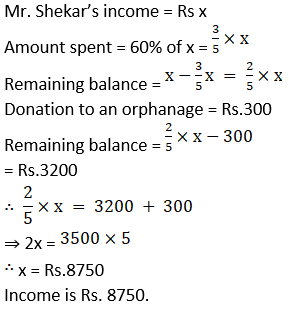3. Mr. Hiralal invested Rs. 2,15,000 in a Mutual Fund. He got Rs. 3,05,000 after 2 years. Mr. Ramniklal invested Rs. 1,40,000 at 8% compound interest for 2 years in a bank. Find out the percent gain of each of them. Whose investment was more profitable?

Solution: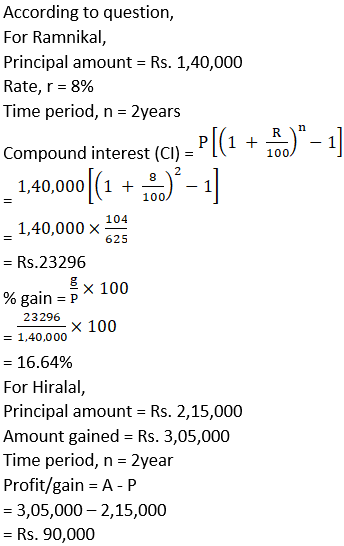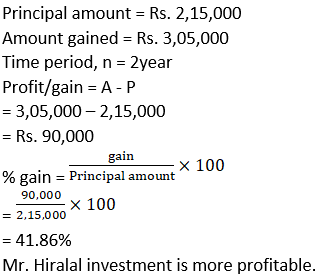4. At the start of a year there were Rs. 24,000 in a savings account. After adding Rs. 56,000 to this the entire amount was invested in the bank at 7.5% compound interest. What will be the total amount after 3 years?

Solution:

Balance at the start of the year = Rs. 24000

Total = Rs. 80,000

Interest rate = 7.5% per annum

Period of accumulation = 3yr

Formula,

A =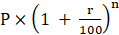Amount of maturity after 3 year,

= Rs.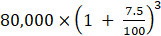= Rs. 80,000×1.075×1.075×1.075

= Rs. 99,383.75

5. Mr. Manohar gave 20% part of his income to his elder son and 30% part to his younger son. He gave 10% of the balance as donation to a school. He still had Rs. 1,80,000 for himself. What was Mr. Manohar’s income?

Solution:

Let the income of Mr. Manohar be ₹ x.

Amount given to elder son = 20% of x

Amount given to younger son = 30% of x

Total amount given to both sons = (20 + 30)% of x = 50% of x

∴ Amount remaining with Mr. Manohar = (100 – 50)% of x

= 50% of x 50

= 50/100 × 100

= 0.5 x

He gave 10% of the balance income as donation to a school.

Amount donated to school = 10% of 0.5x

= 10/100 × 0.5x

= 0.05x

∴ Amount remaining with Mr. Manohar after donating to school = 0.5x – 0.05x

= 0.45x

Mr. Manohar still had 1,80,000 for himself after donating to school.

∴ 180000 = 0.45x

∴ x=180000/0.45

=180000×100/ (0.45×100)

=18000000/45

=400000

∴ The income of Mr. Manohar is ₹4,00,000.

6. Kailash used to spend 85% of his income. When his income increased by 36% his expenses also increased by 40% of his earlier expenses. How much percentage of his earning he saves now?

Solution:

Let Kailash’s salary = Rs. 100

Old spend = 100 × 85% = 85

New salary = 136%of 100 = Rs.136

New expenditure =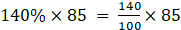= Rs.116

Save = Rs. 136 – Rs. 119

= Rs.17

%S =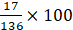= 12.5%

7. Total income of Ramesh, Suresh and Preeti is 8,07,000 rupees. The percentages of their expenses are 75%, 80% and 90% respectively. If the ratio of their savings is 16: 17: 12, then find the annual saving of each of them.

Solution:

Let their savings be 16x,17x,12x

Saving = income – expenditures

Saving% = 100 – expenditure%

Hence saving are 25% ,20%, 10% respectively,

Saving % =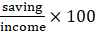Their incomes are,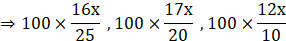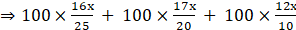= Rs.807000

Then x = Rs. 3000

Hence saving are Rs. 48,000, Rs. 51,000, Rs. 36,000

8. Compute the income tax payable by following individuals.
i. Mr. Kadam who is 35 years old and has a taxable income of Rs. 13,35,000.
ii. Mr. Khan is 65 years of age and his taxable income is Rs. 4,50,000.
iii. Miss Varsha (Age 26 years) has a taxable income of Rs. 2,30,000.

Solution:

(i) In case of Mr. kadam who is 35 years and taxable income is 13,35,000

•up to 250000 – No tax (0 %)

•2,50,000 to 5,00,000 – 12,500 (2,50,000 x 5%)

•5,00,000 to 10,00,000 – 1,00,000 (5,00,000 x 20%)

•remaining 3,35,000 – 1,00,500 (3,35,000 x 30%)

Income Tax is 2,13,000 + Education cess 3% on 2,13,000

Total tax is 2,19,390

(ii) in the case of Mr. Khan who is 65 yrs and 4,50,000 income taxable up to 300000 – No tax (0 %)

remaining 150000 – 7500 (150000 x 5%)

Income Tax is 7500 + Education Cess 3% of 7500

Total Tax is 7725

(iii) In the case of Miss Varsha who is 26 yrs and 2,30,000 taxable income.

Up to 2,50,000 income – no tax

Total tax – NIL

## Frequently Asked Questions on Maharashtra State Board Solutions for Class 9 Maths Part 1 Chapter 6 Financial Planning

### Will these Maharashtra Board Class 9 Solutions be useful to prepare for the exams?

Yes, these solutions can be very helpful. Students are highly recommended to practise these solutions after revising the subjects, as they set the basis for the questions that could get asked often in the board exams.

### How are these Maharashtra State Board Class 9 Maths Part 1 Chapter 6 Solutions helpful?

Students are encouraged to download these solutions and practise them. Then they can refer back to the solutions to analyse their performance. This will also help them to rectify their mistakes and to avoid making them during the board exams.

### Where can we find these Maharashtra State Board Class 9 Solutions ?

Yes, we provide the solutions to download. We have also provided the solutions as a scrollable PDF and we have also mentioned the clickable link for the students to access. Meanwhile, the questions and the solutions  are also available online on our webpage.